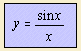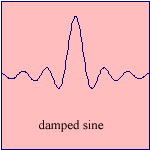# damped sine

## trigonometric

last updated: 2003-12-09where y=1 for x=0.The sine cardinal function (or cardinal sine function) can be seen as an harmonic oscillation for which the amplitude diminishes proportionally with time.
This (non-periodic) damped sine function is also called the sinc function sinc() (being an abbreviation for 'sine cardinal').

Some authors use 2x/p instead of x.

The function is found in the areas of signal processing and Fourier analysis. The function is the Fourier transform of a rectangular pulse, the rectangle function.

The function is equivalent to the spherical Bessel function of the first kind, of order 0: j0().